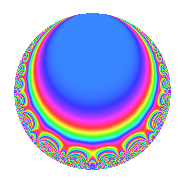# Properties

 Label 18.3.dLevel 18 Weight 3 Character orbit d Rep. character $$\chi_{18}(5,\cdot)$$ Character field $$\Q(\zeta_{6})$$ Dimension 4 Newforms 1 Sturm bound 9 Trace bound 0

# Related objects

## Defining parameters

 Level: $$N$$ = $$18 = 2 \cdot 3^{2}$$ Weight: $$k$$ = $$3$$ Character orbit: $$[\chi]$$ = 18.d (of order $$6$$ and degree $$2$$) Character conductor: $$\operatorname{cond}(\chi)$$ = $$9$$ Character field: $$\Q(\zeta_{6})$$ Newforms: $$1$$ Sturm bound: $$9$$ Trace bound: $$0$$

## Dimensions

The following table gives the dimensions of various subspaces of $$M_{3}(18, [\chi])$$.

Total New Old
Modular forms 16 4 12
Cusp forms 8 4 4
Eisenstein series 8 0 8

## Trace form

 $$4q$$ $$\mathstrut +\mathstrut 4q^{4}$$ $$\mathstrut -\mathstrut 18q^{5}$$ $$\mathstrut -\mathstrut 12q^{6}$$ $$\mathstrut +\mathstrut 2q^{7}$$ $$\mathstrut +\mathstrut 12q^{9}$$ $$\mathstrut +\mathstrut O(q^{10})$$ $$4q$$ $$\mathstrut +\mathstrut 4q^{4}$$ $$\mathstrut -\mathstrut 18q^{5}$$ $$\mathstrut -\mathstrut 12q^{6}$$ $$\mathstrut +\mathstrut 2q^{7}$$ $$\mathstrut +\mathstrut 12q^{9}$$ $$\mathstrut +\mathstrut 18q^{11}$$ $$\mathstrut +\mathstrut 12q^{12}$$ $$\mathstrut -\mathstrut 10q^{13}$$ $$\mathstrut +\mathstrut 36q^{14}$$ $$\mathstrut +\mathstrut 18q^{15}$$ $$\mathstrut -\mathstrut 8q^{16}$$ $$\mathstrut -\mathstrut 24q^{18}$$ $$\mathstrut -\mathstrut 40q^{19}$$ $$\mathstrut -\mathstrut 36q^{20}$$ $$\mathstrut -\mathstrut 42q^{21}$$ $$\mathstrut -\mathstrut 12q^{22}$$ $$\mathstrut +\mathstrut 18q^{23}$$ $$\mathstrut +\mathstrut 4q^{25}$$ $$\mathstrut +\mathstrut 8q^{28}$$ $$\mathstrut +\mathstrut 18q^{29}$$ $$\mathstrut +\mathstrut 72q^{30}$$ $$\mathstrut +\mathstrut 38q^{31}$$ $$\mathstrut +\mathstrut 54q^{33}$$ $$\mathstrut +\mathstrut 24q^{34}$$ $$\mathstrut +\mathstrut 12q^{36}$$ $$\mathstrut +\mathstrut 128q^{37}$$ $$\mathstrut -\mathstrut 72q^{38}$$ $$\mathstrut -\mathstrut 102q^{39}$$ $$\mathstrut -\mathstrut 126q^{41}$$ $$\mathstrut -\mathstrut 48q^{42}$$ $$\mathstrut -\mathstrut 46q^{43}$$ $$\mathstrut -\mathstrut 54q^{45}$$ $$\mathstrut -\mathstrut 24q^{46}$$ $$\mathstrut +\mathstrut 54q^{47}$$ $$\mathstrut +\mathstrut 24q^{48}$$ $$\mathstrut -\mathstrut 12q^{49}$$ $$\mathstrut -\mathstrut 72q^{51}$$ $$\mathstrut +\mathstrut 20q^{52}$$ $$\mathstrut +\mathstrut 36q^{54}$$ $$\mathstrut -\mathstrut 108q^{55}$$ $$\mathstrut +\mathstrut 72q^{56}$$ $$\mathstrut +\mathstrut 144q^{57}$$ $$\mathstrut +\mathstrut 24q^{58}$$ $$\mathstrut +\mathstrut 126q^{59}$$ $$\mathstrut -\mathstrut 36q^{60}$$ $$\mathstrut +\mathstrut 62q^{61}$$ $$\mathstrut +\mathstrut 222q^{63}$$ $$\mathstrut -\mathstrut 32q^{64}$$ $$\mathstrut +\mathstrut 90q^{65}$$ $$\mathstrut -\mathstrut 72q^{66}$$ $$\mathstrut -\mathstrut 106q^{67}$$ $$\mathstrut +\mathstrut 72q^{68}$$ $$\mathstrut +\mathstrut 18q^{69}$$ $$\mathstrut -\mathstrut 108q^{70}$$ $$\mathstrut -\mathstrut 96q^{72}$$ $$\mathstrut -\mathstrut 208q^{73}$$ $$\mathstrut +\mathstrut 72q^{74}$$ $$\mathstrut -\mathstrut 12q^{75}$$ $$\mathstrut -\mathstrut 40q^{76}$$ $$\mathstrut -\mathstrut 90q^{77}$$ $$\mathstrut +\mathstrut 144q^{78}$$ $$\mathstrut +\mathstrut 14q^{79}$$ $$\mathstrut -\mathstrut 252q^{81}$$ $$\mathstrut +\mathstrut 144q^{82}$$ $$\mathstrut -\mathstrut 378q^{83}$$ $$\mathstrut -\mathstrut 144q^{84}$$ $$\mathstrut +\mathstrut 108q^{85}$$ $$\mathstrut -\mathstrut 108q^{86}$$ $$\mathstrut -\mathstrut 54q^{87}$$ $$\mathstrut +\mathstrut 24q^{88}$$ $$\mathstrut +\mathstrut 412q^{91}$$ $$\mathstrut +\mathstrut 36q^{92}$$ $$\mathstrut +\mathstrut 222q^{93}$$ $$\mathstrut +\mathstrut 84q^{94}$$ $$\mathstrut +\mathstrut 180q^{95}$$ $$\mathstrut +\mathstrut 48q^{96}$$ $$\mathstrut +\mathstrut 14q^{97}$$ $$\mathstrut +\mathstrut 126q^{99}$$ $$\mathstrut +\mathstrut O(q^{100})$$

## Decomposition of $$S_{3}^{\mathrm{new}}(18, [\chi])$$ into irreducible Hecke orbits

Label Dim. $$A$$ Field CM Traces $q$-expansion
$$a_2$$ $$a_3$$ $$a_5$$ $$a_7$$
18.3.d.a $$4$$ $$0.490$$ $$\Q(\sqrt{-2}, \sqrt{-3})$$ None $$0$$ $$0$$ $$-18$$ $$2$$ $$q+\beta _{1}q^{2}+(1-2\beta _{1}-2\beta _{2}+\beta _{3})q^{3}+\cdots$$

## Decomposition of $$S_{3}^{\mathrm{old}}(18, [\chi])$$ into lower level spaces

$$S_{3}^{\mathrm{old}}(18, [\chi]) \cong$$ $$S_{3}^{\mathrm{new}}(9, [\chi])$$$$^{\oplus 2}$$